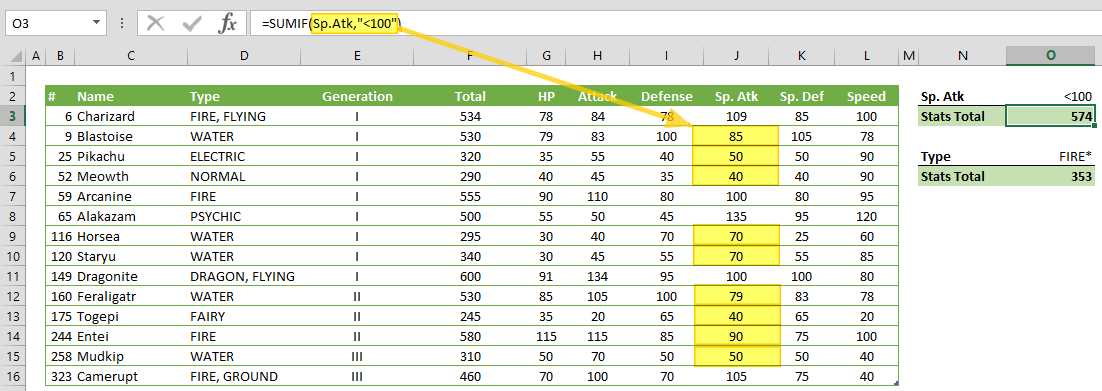SUMIF function can sum up the values in a range of cells that match a single criteria. In this guide, we’re going to show you how to use the SUMIF function and also go over some tips and error handling methods.

# Supported versions

• All Excel versions

# Syntax

SUMIF(range, criteria, [sum_range])

# Arguments

 range The range of cells that you want to apply the criteria against. criteria The criteria that is applied to range to define which cells to add. sum_range Optional. The range of cells to sum.

Examples

We're going to be using named ranges our examples. You don't have to do this, we just added them to make it easier to read the formulas.

## Example 1

=SUMIF(Sp.Atk,"<100")
formula adds Sp.Atk values where Sp.Atk is less than 100. Since both the criteria and the sum ranges are the same, the sum_range argument can be omitted from the SUMIF formula.## Example 2

=SUMIF(Type,"FIRE*",HP)
formula sums up the HP values, where the Type starts with the specified string "FIRE". If we only use "FIRE" without the asterisks, the SUMIF function would skip the HP values for "FIRE, GROUND" and "FIRE, FLYING".

Note: SUMIF function is not case-sensitive. So, "FIRE" and "fire" will yield the same results.# Tips

• Use the same number of rows and columns for sum and criteria range arguments.
• Good Example: =SUMIF(F2:F11,">2014",G2:G11)
• Comparison operators:
 Operator Description Criteria Sample Criteria Meaning = Equal to “=10000” Equal to 10000 <> Not equal to “<>10000” Not equal to 10000 > Greater than “>10000” Greater than 10000 < Less than “>10000” Less than 10000 >= Greater than or equal to “>=10000” Greater than or equal to 10000 <= Less than or equal to “<=10000” Less than or equal to 10000 ? Takes the place of a single character “Admin?” 6-character word starts by “Admin” * Can take the place of any number of characters. “Admin*” Any number of character word starts with “Admin” ~ Use tilde in front of a  question mark or an asterisk to actually find them “Admin~*” Equal to "Admin*"

Note: Wildcards cannot be used for numeric values. Searching a wild card in a range of numeric values will returns no matches.

# Issues

## #VALUE!

The SUMIF function returns incorrect results when you use it to match strings longer than 255 characters, or with the string #VALUE!.

## TRUE and FALSE

TRUE and FALSE values in sum_range are evaluated as numbers. While TRUE is evaluated as 1, FALSE is evaluated as 0. As a result, this condition can yield unexpected results when added like a cell value.